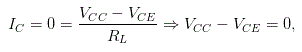# MCQs on Analog Electronics

##### Page 32 of 33. Go to page 1 2 3 4 5 6 7 8 9 10 11 12 13 14 15 16 17 18 19 20 21 22 23 24 25 26 27 28 29 30 31 32 33
01․ When there is no base current in a transistor switch, the output voltage from the transistor is
low.
high.
unchanged.
unknown.

In a transistor, if the base current is also zero, then the collector current is also zero. In a transistor, collector currentwhich gives VCC = VCE. Hence, the output voltage from the transistor is highs.

02․ The first step in analyzing emitter-based circuits is to find the
base current.
emitter current.
supply current.
collector current.

The first step in analyzing the emitter based circuit is to find the emitter current. This is given clearly in the below analysis of emitter biased circuit.

03․ If the current gain is unknown in an emitter-biased circuit, you cannot calculate the
emitter voltage.
emitter current.
collector current.
base current.

In an emitter-biased circuit, the emitter current (IE) = current gain (β) X base current (IB). So, if we don't know the current gain in an emitter biased circuit, we can't calculate the base current.

04․ If the emitter resistor is open, the collector voltage is
low.
high.
unchanged.
unknown.

If the emitter resistor is open, then the full supply voltage is going to the output load because the current chooses the low resistance path to flow and so it does not flow through the emitter resistance. Hence, the collector voltage is high.

05․ If the collector resistor is open, the collector voltage is
low.
high.
unchanged.
unknown.

If the collector resistor is open, then there is no way to flow the current to load. Hence, the output voltage is low at load side.

06․ When the current gain increases from 50 to 300 in an emitter-biased circuit, the collector current
remains almost the same.
decreases by a factor of 6.
increases by a factor of 6.
is zero.

In an emitter biased circuit, there is no relation between collector current and the current gain. Hence, it remains almost the same.

07․ If the emitter resistance increases, the collector voltage
decreases.
stays the same.
increases.
breaks down the transistor.

If the emitter resistor increases, then the most of the supply voltage is going to the output load because the current chooses the low resistance path to flow and so it does not flow through the emitter resistance. Hence, the collector voltage also increases.

08․ If the emitter resistance decreases, the
Q point moves up.
collector current decreases.
Q point stays where it is.
current gain increases.

If the emitter resistor decreases, then the flow of collector current is more because the current chooses the low resistance path to flow. Hence, the current gain also increases. That means the Q point moves up from the previous level.

09․ When testing an npn transistor using an ohmmeter, the collector-emitter resistance will be low when
the collector is positive in respect to the emitter.
the emitter is positive in respect to the collector.
the transistor is normal.
the transistor is defective.

The collector-emitter resistance is nothing but the output impedance. Typically, for an NPN transistor, the output impedance is around 100000 Ω. So, when testing an NPN transistor if it shows the low output impedance, then that means the transistor is faulty.

10․ The major advantage of a photo transistor as compared to a photo diode is its
response to higher frequencies.
AC operation.
increased sensitivity.
durability.

Most phototransistors have outer casing which is either transparent or has a clear lens to focus the light onto the base junction for increased sensitivity. Also, in phototransistor, the current gain is too high and this is nothing but the sensitivity of a phototransistor. But this is not available in photo diodes. That is why the sensitivity of the photo transistor is greater than the photo diode.

<<<30313233>>>